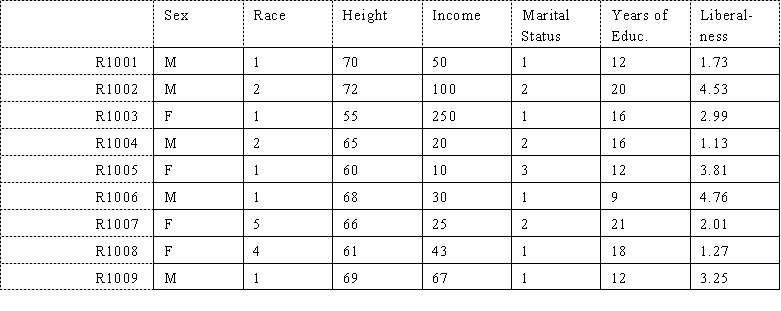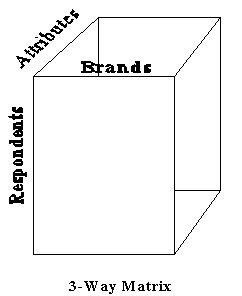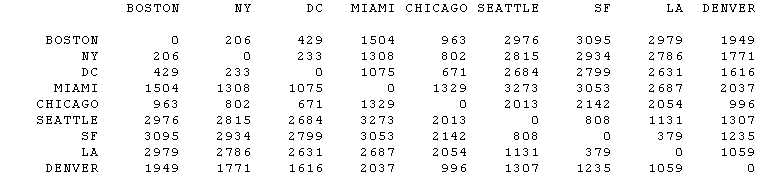Matrices
by Steve Borgatti, Boston College

Overview

The typical dataset collected by a sociologist and certain kinds of anthropologists can be formatted as a matrix (see figure) in which the rows correspond to respondents and the columns correspond to variables. For example:This kind of matrix is called "2-way" because it is 2-dimensional: it has both rows and columns. A one-way matrix is just a single list of numbers -- it would ordinarily be called an array or a vector.Some matrices are 3-way, because they have rows, columns and levels. For example, if we ask ten respondents to rate 3 brands of computer on 5 attributes, the result would be a 3-way matrix, which we can think of as a 3-dimensional object, like a cube (see figure). Alternatively, we can think of the data as forming a collection of 2-way matrices, each of which is a slice from the cube. For example, we might consider the data to consist of five respondent-by-brand matrices, one for each attribute. These correspond to vertical slices of the cube. Another way to think of it is that each respondent is rating each brand on every attribute, so there is a brand-by-attribute matrix for each respondent. This corresponds to taking horizontal slices through the cube.

If we then collected the same data on the same respondents at different points in time, we could record the whole thing in a 4-way matrix, which we can think of as a collection of cubes, one for each time period.

Note that in our original 2-way matrix, the rows and columns "point to" different kinds of entities. The rows represent persons and the columns represent variables. This is not always the case. For example, the following matrix of driving distances between U.S. cities has cities as both the rows and the columns:Each distinct type of entity referenced by the ways of a matrix is known as a mode. The original respondent-by-variable matrix had two modes. The matrix of driving distances has one mode. The data cube shown above has three modes. Suppose we measure driving distances between pairs of cities before and after a great deal of new road construction. Such data would form a 3-way 2-mode city-by-city-by-time matrix.

In general, relationships among a set of objects form matrices with fewer modes than ways, while relationships between sets of objects form matrices with as many modes as ways. For example, if we record who is a friend of whom among the members of an organization, the resulting matrix is 2-way, 1-mode. If we record which faculty would like to teach which courses next semester, the resulting matrix would be 2-way, 2-mode.

It should be noted that a matrix is an abstract, mathematical object which is independent of how we actually format data. For example, the matrix of distances could be recorded as a list of unordered pairs, as follows:Note that we have not bothered to record that the distance from NY to BOSTON was also 206, since we knew the matrix was symmetric. Most matrices that represent physical proximities or similarities are symmetric. We have also not recorded the distance of a city to itself, since that is zero by definition.

Useful References

Mathematical

• Perlis, S. 1991. Theory of Matrices. Dover.

Statistical

• Green, P.E. 1976. Mathematical Tools for Applied Multivariate Analysis. Academic Press. [a terrific book, but difficult]

Data Theory

• Jacoby. Data Theory and Dimensional Analysis. Sage.
• Borgatti, S. P. 1994. Cultural domain analysis. Journal of Quantitative Anthropology, 4: 261-278. [pdf]